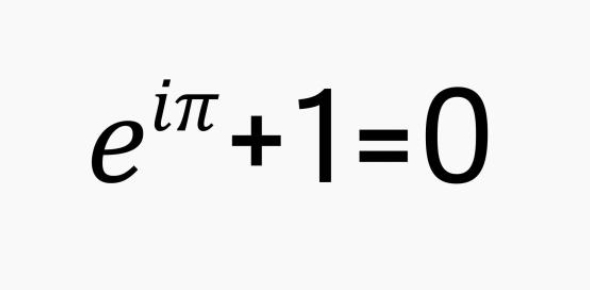# Pi Trivia Quiz

10 Questions | Attempts: 2940
ShareSettingsPi—is a symbol in mathematics that is written as the Greek letter for p or π. The approximate value of this important symbol in mathematics is 3. 141592. Pi denotes the ratio of a circle's circumference to its diameter. Pi was found by Archimedes of Syracuse. It has its origin in ancient Babylonian civilization. How well aware are you about this magical number? Play this exciting quiz to test your knowledge about Pi. This Pi trivia quiz is best played with friends and family for more adventure! Learning made easy and fun!

• 1.
Who, in 1706, first gave the Greek letter “pi” its current mathematical definition?
• A.

Albert Einstein

• B.

William Jones

• C.

Attila the Hun

• D.

Archimedes

• E.

Napoleon Bonaparte

• 2.
Pi is transcendental.  What does this mean, in mathematics?
• A.

It is equal to the ratio of two integers

• B.

Its square root is imaginary

• C.

It cannot be expressed as an integer, or as a root or quotient of integers

• D.

It was Ralph Waldo Emerson’s favorite number.

• 3.
What is the earliest known reference to pi in history?
• A.

The Rosetta Stone, approx. 200 BC

• B.

The Bible

• C.

An Egyptian papyrus scroll, written approx. 1650 BC by Ahmes the Scribe

• D.

Euclid’s Elements, written in the 3rd century BC

• 4.
People tried for centuries to “square the circle”.  What were they trying to do?
• A.

Construct a square that perfectly circumscribes (surrounds) a given circle

• B.

Determine the value of pi squared

• C.

Multiply a circle by itself

• D.

Use a straightedge and compass to construct a square exactly equal in area to a given circle

• E.

Alter a recipe intended for a round pan so that it would fit exactly into a square pan

• 5.
Some people became mentally deranged when trying to “square the circle”.  What was this illness named?
• A.

Impossibilius Fittus

• B.

Morbus Cyclometricus

• C.

Repetitionatis Decimalus

• 6.
Are pi’s digits periodic?  In other words, do the digits ever repeat themselves in any pattern?
• A.

Yes. The digits repeat themselves every 6,000,000 decimal places

• B.

No. Every periodic number is rational, but pi is irrational

• C.

Yes. Every infinitely long number repeats itself

• D.

Perhaps. Not enough digits of pi have been calculated to know yet

• 7.
Pi is an irrational number.  What does that really mean?
• A.

Its digits cannot be rationed out evenly

• B.

Nobody with sound judgement has anything to do with it

• C.

It is a real number, but can’t be expressed as a ratio of two integers

• 8.
Among the digits of pi currently known, the concentration of each of the digits 0-9 are pretty close to equal.  However, in the first 30 places of pi’s decimal expansion, which digit is completely missing?
• A.

7

• B.

2

• C.

0

• D.

8

• 9.
What is the “formal” definition of pi?
• A.

The surface area of a sphere of diameter 22/7

• B.

3.1415926

• C.

• D.

A delicious dessert

• E.

The ratio of a circle’s circumference to its diameter

• 10.
Imagine if you wrapped a rope tightly around the earth at the equator.  How much longer would you have to make the rope if you wanted it to be exactly one foot above the surface all the way around?
• A.

2 ∏ feet

• B.

2 ∏ r feet, where r is the radius of the earth

• C.

∏ r ^2 feet

## Related TopicsBack to top
×

Wait!
Here's an interesting quiz for you.